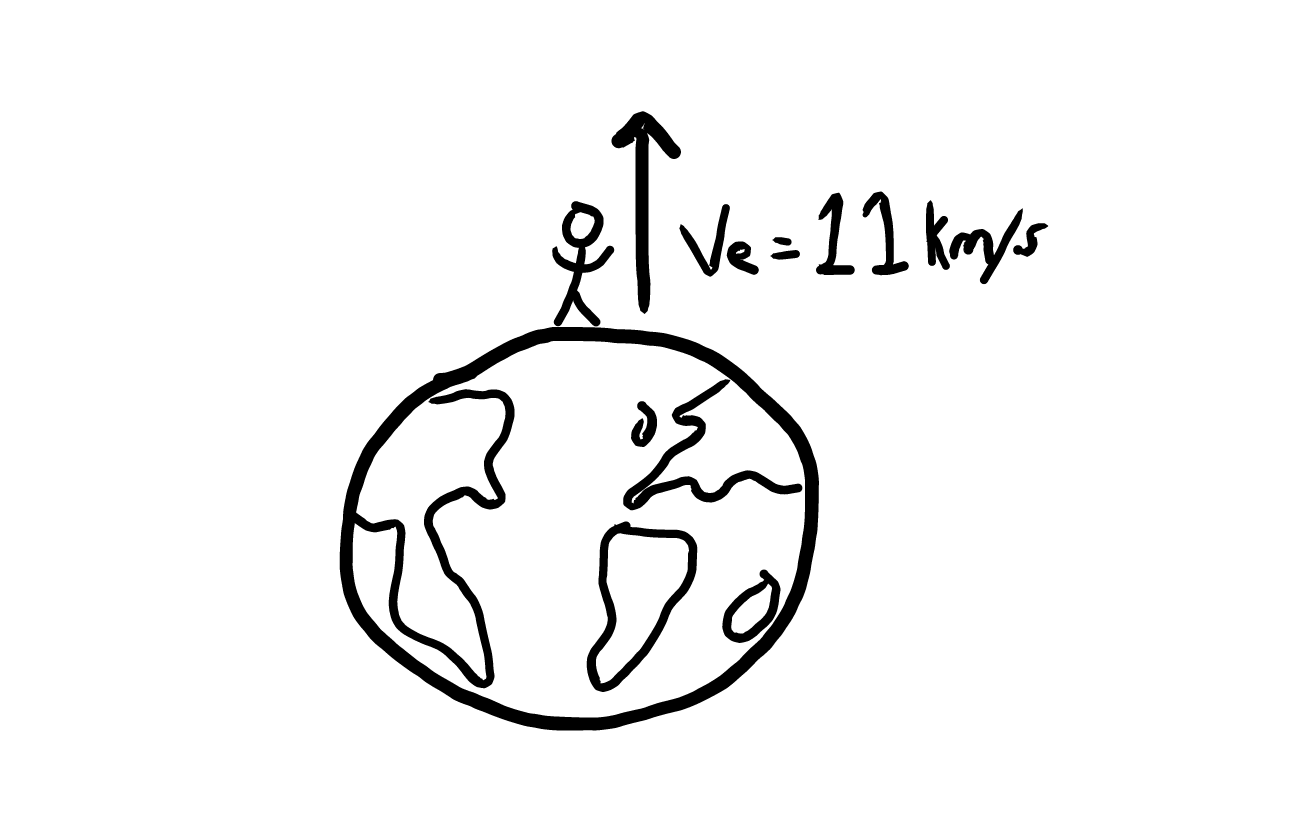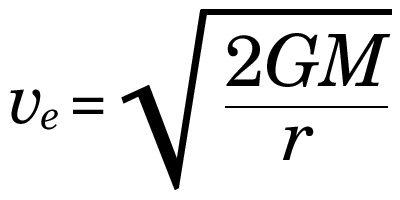# Physics : Gravitation -2

## Kepler’s Laws of  Planetary motion

1. Law of orbit – Every planet revolve around the sun in elliptical orbit and sun is at its one focus.1. Law of Areal Velocity : – A planet moves round the sun in such a way that its areal velocity always remains constant.Area of ABM = Area of CDM = Area of EFM

• Areal velocity is the state at which area “ i ” sweat out by a particle as it moves along a curve
• Second law can also defined as –

“The line joining the sun and the planet sweeps out equal areas in equal times.

1. Law of period: – A planet moves round the sun in such a way that the square of its period is proportional to the cube of semi major axis of its elliptical orbit.Escape Velocity (Ve)

It is the minimum velocity with which a body has to be projected vertically upwards from the earth, so that it just crosses the earth’s gravitational field and never returns.Escape VelocityDid you know?

• Escape velocity does not depends on mass, shape and size of the body.
• I is also called second cosmic velocity
• Escape velocity of earth = 11.2 km/s
• Escape velocity of Moon = 2.4 km/s

Satellite:- Satellites are natural or artificial bodies revolving around a planet under its gravitational attraction.

• Moon is the natural satellite, while INSAT-1B is an artificial satellite of earth.

Orbital velocity of a satellite

• It is the minimum velocity required to place or maintain satellite into a given orbit around earth.Where, M = Mass of the planet

r = Radius of the Planet

H = height of the satellite from planet’s surface.

Planet’s surface.

Previous year important questions

Q.1       What is the approximate height of any geostationary satellite from earth’s surface?

(a) 36000 km                (b) 45000 km                (c) 50000 km                (d) 25000 km

Ans. (a) Geostationary satellite is placed in the orbit which is at an altitude of 36000 km (Approximately) above mean sea level.

Q.2      The spoon dropped by an astronaut in a satellite will –

(a) Fall to the floor

(b) Remain stationary

(c) Continue to follow the motion of the satellite

(d) Move tangentially away.

Ans. (c)            Since, the spoon is inside the satellite it will acquire the velocity of satellite hence, on dropping, it will keep moving with the satellite velocity.

Q.3       What is the minimum escape velocity of rocket to be launched into space?

(a) 5 km/sec                  (b) 6 km/sec                  (c) 11 km/sec                (d) 15 km/sec.

Ans. (c)

Q.4       If the orbit of a planet is an ellipse then what is the point at which the sun is located called?

(a) Centre                     (b) Circum centre          (c) In centre                  (d) Focus

Ans. (d) Due to the force of gravity, planet trace out an ellipse in space as they orbit around the sun which is located at a single focus.

#### You may also like...

This site uses Akismet to reduce spam. Learn how your comment data is processed.